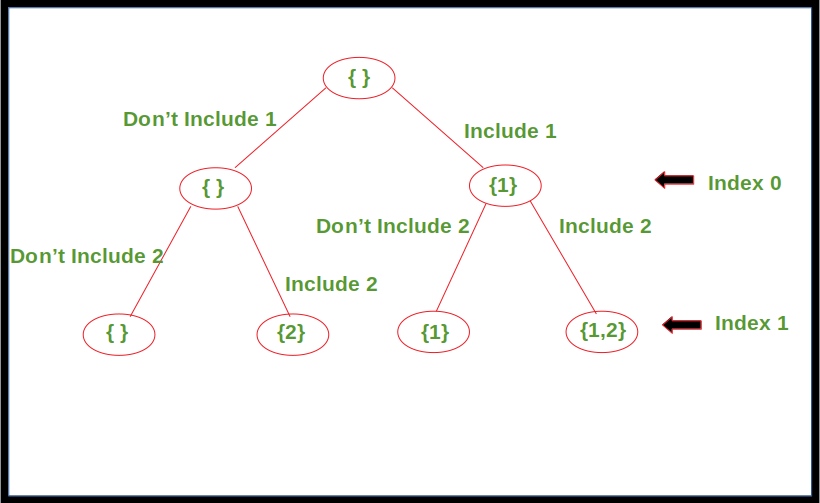# Generating all possible Subsequences using Recursion

## Generating all possible Subsequences using Recursion

Given an array. The task is to generate and print all of the possible subsequences of the given array using recursion.

Examples:

```Input : [1, 2, 3]
Output : , , [2, 3], , [1, 3], [1, 2], [1, 2, 3]

Input : [1, 2]
Output : , , [1, 2]
```

Approach: For every element in the array, there are two choices, either to include it in the subsequence or not include it. Apply this for every element in the array starting from index 0 until we reach the last index. Print the subsequence once the last index is reached.

Below diagram shows the recursion tree for array, arr[] = {1, 2}.Below is the implementation of the above approach.

 `// C++ code to print all possible` `// subsequences for given array using` `// recursion` `#include ` `using` `namespace` `std;` `void` `printArray(vector<``int``> arr, ``int` `n)` `{` `    ``for` `(``int` `i = 0; i < n; i++)` `        ``cout << arr[i] << ``" "``;` `    ``cout << endl;` `}` `// Recursive function to print all` `// possible subsequences for given array` `void` `printSubsequences(vector<``int``> arr, ``int` `index,` `                       ``vector<``int``> subarr)` `{` `    ``// Print the subsequence when reach` `    ``// the leaf of recursion tree` `    ``if` `(index == arr.size())` `    ``{` `        ``int` `l = subarr.size();` `        ``// Condition to avoid printing` `        ``// empty subsequence` `        ``if` `(l != 0)` `            ``printArray(subarr, l);` `    ``}` `    ``else` `    ``{` `        ``// Subsequence without including` `        ``// the element at current index` `        ``printSubsequences(arr, index + 1, subarr);` `        ``subarr.push_back(arr[index]);` `        ``// Subsequence including the element` `        ``// at current index` `        ``printSubsequences(arr, index + 1, subarr);` `    ``}` `    ``return``;` `}` `// Driver Code` `int` `main()` `{` `    ``vector<``int``> arr{1, 2, 3};` `    ``vector<``int``> b;` `    ``printSubsequences(arr, 0, b);` `    ``return` `0;` `}` `// This code is contributed by` `// sanjeev2552`

Output:

```

[2, 3]

[1, 3]
[1, 2]
[1, 2, 3]```

Time Complexity:Last Updated on March 1, 2022 by admin

## Python – Itertools.chain()Python – Itertools.chain()

Python – Itertools.chain() The itertools is a module in Python having a collection of functions that are

## Comprehensions in PythonComprehensions in Python

Comprehensions in Python Comprehensions in Python provide us with a short and concise way to

## Python – Sentiment Analysis using VADERPython – Sentiment Analysis using VADER

Python | Sentiment Analysis using VADER Sentiment Analysis is the process of ‘computationally’ determining whether a

## Python – Coefficient of Determination-R2 scorePython – Coefficient of Determination-R2 score

Python – Coefficient of Determination-R2 score Coefficient of determination also called as R2 score is used

## Get directory of current Python scriptGet directory of current Python script

Get directory of current Python script While working with file handling you might have noticed that files

## Saving a Video using OpenCVSaving a Video using OpenCV

Saving a Video using OpenCV OpenCV is an open-source and most popular computer vision library

## Q-Learning in PythonQ-Learning in Python

Q-Learning in Python Reinforcement Learning briefly is a paradigm of Learning Process in which a learning

## get_attribute() element method – Selenium Pythonget_attribute() element method – Selenium Python

get_attribute() element method – Selenium Python get_attribute() element method – Selenium Python Difficulty Level : Basic